﻿ A Simple Current Control Strategy for Single-Stage Grid Connected Three-Phase PV Inverter

A Simple Current Control Strategy for Single-Stage Grid Connected Three-Phase PV Inverter

P. K. Hota, Babita Panda, Bhagabat Panda

American Journal of Electrical and Electronic Engineering

A Simple Current Control Strategy for Single-Stage Grid Connected Three-Phase PV Inverter

P. K. Hota1,, Babita Panda2, Bhagabat Panda2

1Department of Electrical Engineering, Veer Surendra Sai University of Technology, Burla, India

2School of Electrical Engineering, KIIT University, Bhubaneswar, India

Abstract

This paper presents a new simple method of current control strategy of grid connected PV system. As the solar irradiation is a nonlinear quantity, so the connection of PV system with the grid is a difficult task. The objective of this work is to develop a model of the photovoltaic system with MPPT connected to 11KV grid by implementing new control technique so that maximum active power transfer from PV inverter to grid can be taken place without injection of harmonics. This paper also demonstrates the dynamic model of single-stage three-phase grid connected inverter. Here, for simplification the PV system is realized as a constant DC voltage source by using maximum power point tracking (MPPT) and boost converter. A current control strategy with pulse width modulation (PWM) technique is proposed to provide pulse for voltage-source inverter (VSI). The analysis and control design of grid connected PV inverter using PI control technique is done in synchronous d-q rotating reference frame to achieve maximum output voltage response and active power. The considered system consists of a VSI, 3-Φ filter, a control system, a distribution network, load and grid. As PV inverter should inject only active power, so reactive power injected to the grid is made zero with the help of this control technique. There after the final model is simulated by using MATLAB/SIMULINK and different output waveforms are analyzed for three different conditions.

• P. K. Hota, Babita Panda, Bhagabat Panda. A Simple Current Control Strategy for Single-Stage Grid Connected Three-Phase PV Inverter. American Journal of Electrical and Electronic Engineering. Vol. 4, No. 4, 2016, pp 102-109. http://pubs.sciepub.com/ajeee/4/4/1
• Hota, P. K., Babita Panda, and Bhagabat Panda. "A Simple Current Control Strategy for Single-Stage Grid Connected Three-Phase PV Inverter." American Journal of Electrical and Electronic Engineering 4.4 (2016): 102-109.
• Hota, P. K. , Panda, B. , & Panda, B. (2016). A Simple Current Control Strategy for Single-Stage Grid Connected Three-Phase PV Inverter. American Journal of Electrical and Electronic Engineering, 4(4), 102-109.
• Hota, P. K., Babita Panda, and Bhagabat Panda. "A Simple Current Control Strategy for Single-Stage Grid Connected Three-Phase PV Inverter." American Journal of Electrical and Electronic Engineering 4, no. 4 (2016): 102-109.

 Import into BibTeX Import into EndNote Import into RefMan Import into RefWorks

123456
Prev Next

1. Introduction

In recent years, as the demand for the need of electric power is increasing, the research regarding grid connected PV system has increased rapidly. Many research papers have been published on this technology considering various control strategies for three-phase systems. Amongst others, Blaabjerg, et al  have proposed specification and requirement of interconnection of DG with existing power system. The authors have presented an overview of control and grid synchronization for distributed power generation systems. The inverter is the key component for the successful operation of grid connected PV system. Generally PWM techniques are applied to control the performance of Inverter. Ho, et al  have described the implementation of hysteresis current control technique to track the reference sinusoidal current. A comparative study of current controlled PWM and open loop PWM has been described by Tekwani, et al . Blaabjerg, et al  have analyzed the advantages and limitations of interfacing power electronic equipment like inverter with the grid by using MATLAB. Schonardie, et al  have applied dq0 transformation on the three-phase grid connected PV system with active power control. Advantages of different current control techniques and implementation of PI controller is described by Rahman, et al . Kazmier, et al  have described a survey on PWM current control techniques of voltage source converters. A control methodology has been proposed by Yazdani, et al  and characterization of dynamics for a photovoltaic system interfaced with a distribution network has been made. Krismadinata, et al  have described the procedure implementation of hysteresis current control for single-phase grid connected inverter. Habeebullah, et al  have proposed a new control paradigm for integration of photovoltaic energy sources with utility network. Xunjiang, et al  have described the research of photovoltaic grid-connected inverter based on adaptive current hysteresis band control scheme. Many previous works have investigated different control aspects of inverter that is connected to grid. Most of the analysis is done to reduce total harmonic distortion (THD) of the system.

The main aim of this study is to co-ordinate the voltage, active power as well as reactive power injected to grid which is not analysed in the previous papers. The proposed work gives the information about a three-phase PWM inverter modelling and its control strategy. Dynamic modelling and simulation of a single-stage grid connected inverter have been carried out in this paper. For simplicity, PV system is realized as a constant DC voltage source by using maximum power point tracking (MPPT) and a boost converter. Particularly, the control design of grid connected inverter using PI control technique has been proposed in d-q rotating frame in order to achieve maximum output voltage and maximum active power. A simple current control strategy with PWM technique has been proposed to provide pulse for voltage source inverter (VSI). The proposed model is simulated in MATLAB/SIMULINK environment and the obtained different output waveforms for three different conditions are analysed.

The paper is organized as follows. The current control strategies in grid connected system are given in Section 2. Section 3 is dedicated to simulation results and analysis, followed by conclusion in Section 4 and references in Section 5.

2. VSI and Its Control Technique

Broadly inverters are of two types according to voltage and current requirements in the load end.

i) CSI (current source inverter)

The semiconductor devices like a gate-turn-off thyristor (GTO) or silicon controlled rectifier (SCR) or a power transistor with a series diode with reverse blocking capability are used in CSI. At least one of the lower leg devices has to be gated on and maintained on at any time. Otherwise, an open circuit of the dc inductor would occur and destroy the devices. The open-circuit problem by Electro Magnetic Interference (EMI) noise’s misgating-OFF state is a major concern of the converter’s reliability. Overlap time for safe current commutation is needed in the current-source converter, which also causes waveform distortion, etc.

ii) VSI (voltage source inverter)

A power transistor and an antiparallel (or free-wheeling) diode are used to provide bidirectional current flow and unidirectional voltage blocking capability. At one time the upper and lower devices of each phase leg cannot be gated on. Otherwise, a shoot-through would occur and destroy the devices. An output LC filter is needed for providing a sinusoidal voltage compared with the current-source inverter, which causes additional power loss and control complexity.

Except some specific load most of the application require constant voltage supply and hence, VSI is more often used and well-known. Grid connected 3-Φ inverters are normally VSIs. It can provide constant voltage in output end and maintain the load torque.

PV panel is connected to the AC grid which is maintained at 11KV via a common DC/AC inverter. Inverter must produce higher voltage than the grid to assure power flow to the grid. So, PV inverter needs more attention from control point of view. PWM techniques are used to provide the gating pulses for the switches in each leg of the inverter. The aim of the control strategy is to regulate the current output from the inverter to follow a specified reference signal. The most practicable way of doing this is realized by converting the three-phase output currents of the inverter to the rotating reference frame (dq0) and then again converting them to the desired value. Here, the system is assumed to be a balanced system. So, by using Park’s transformation theory the abc components of three-phase currents injected by the inverter are converted into three constant DC components that are defined as the direct, quadrature and zero components: Id, Iq and I0, respectively. From this dq transformation, the constant DC quantities are obtained as shown in equation (1) which can be easily controlled by using proportional-integral (PI) controllers .(1)

By using the above equation, the transformation between the natural (abc) and rotating (dq) frames is done where, T is the transformation matrix. Real and reactive powers injected by the inverter to the grid can be calculated in rotating frame using the following equations .(2)(3)

Where, P is the active power injected to grid, Q is the reactive power injected to grid. Vd, Vq, Id and Iq are the dq voltages and currents at the point of common coupling (PCC). A phase locked loop (PLL) is used to synchronize with the grid frequency. As PV should only injects active power to the grid, so by putting Vq=0, decoupling of P and Q can be done as given below.(4)(5)

Which means that since the grid voltage is uncontrollable, the most practicable way of controlling the operation of the system is by controlling Id and Iq that are flowing to the grid. As active power is dependent on the current Id, so to inject real power to the grid, Id must be regulated to follow a specified reference signal Idref and reactive power injection to the grid is set to zero and thus, Iqref = 0. By using PI controller Idref can be extracted from the power balance equation as given below.(6)

by putting Vq=0,the equation (6) becomes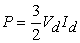(7)

or,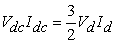(8)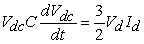(9)

Where, C is the DC link capacitance at the input of the inverter,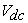is DC link voltage,is current at the DC or input side.

Taking Laplace transform on both the sides,(10)

Where,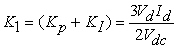(11)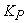is the proportional gain,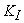is the integral gain of PI compensator.

This was tuned to get the parameters of PI controller.

The PI controller processes the error signal with the help of its parameters. In the proposed model, two PI controllers are used. The output of a controller that processes the error signal is given by the following equation.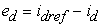(12)

Similarly, the output of other controller is given by:(13)

From the d-q transformation the constant DC quantities are obtained which can be easily controlled by this proportional-integral (PI) controllers. The PI controller constants are chosen as: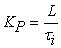(14)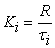(15)

Where,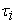is the time constant of the current control loop. The switching frequency of the inverter is 2 kHz and the time constantis set to be ten times smaller than the switching time. Thevalue is chosen as 0.5 andis calculated as 10. Then the PWM modulating signals are generated by transformation of d and q signals into a, b, c and the gating pulses for the VSC valves are sent out. By controlling the values md and mq, the id and iq rapidly track their respective reference commands idref and iqref. In the given control scheme because of the control action, the output current of the inverter follows a specified reference signal. This is achieved by transforming the three-phase output currents of the inverter to the rotating reference frame (dq0) and then again convert them to the desired value. In accordance with IEEE recommended practice for utility interface of PV system (IEEE Standards 929-2000), mostly the PV inverters designed for utility-interconnected service operate close to unity power factor. For a unity power factor, the command Iqref is set to zero.

3. Simulation Results and Analysis

The Figure 1 given below illustrates the block diagram of grid connected inverter. The total system consist of three phase inverter, sinusoidal filter, step-up delta/star transformer, distribution network, phase locked loop, dq-abc transformation block, PWM generator, distribution network, load and grid. Three phase Inverter converts 1500 V of constant DC voltage into sinusoidal A.C voltage, then a step-up delta/star transformer is used to boost voltage up to 11 KV. A phase locked loop (PLL) is used to track the phase angle which is required by the dq-abc transformation block. Again the signal is transformed back to abc reference frame and that is fed to the PWM generator which provides pulse for grid connected PV inverter.Download asVeiw figureFigures index
Figure 1. Block diagram of the proposed system

The Figure 2(a) depicts about the simulink model of grid connected inverter. The total system is simulated in MATLAB/simulink environment. At the input side a constant D.C voltage of magnitude 1500V is used, which in future can be generated by using PV, MPPT and boost converter combination. The three phase voltage source Inverter is simulated by using 3-arm universal bridge, where IGBT with antiparallel diodes are used as power electronic switches. Here, designing of filter is eliminated. A three-phase two winding ∆/Ƴ transformer is used to step-up the voltage up to grid voltage that is 11KV. Grid is analyzed by using a three-phase programmable voltage source having Vrms phase-to- phase 11KV voltage. Three-phase voltage and current measurement units are used to measure voltage and current either in per unit values or in volts and amperes. Here, the voltage and currents measurement are taken in p.u system to normalize the system. Serious errors are avoided by using per unit system during calculations, while referring quantities from one side of the transformer to the other side. Voltages, currents and impedances expressed in per unit do not change during the operation and they lie within a narrow range, which makes calculation easier.

The Figure 2(b) depicts about simulink model of the current control strategies of grid connected inverter. The current control strategy is mainly based on d-q reference frame. The measurement unit tracks Vabc and Iabc that is voltage and current of the grid. By using abc-dq transformation block, Vabc and Iabc can be converted into Vd, Vq, Id and Iq. The controller block performs control action. Then by comparing Id, Iq with Idref and Iqref, the error signals are generated.Download asVeiw figureFigures index
Figure 2 (a). Simulink model of grid connected inverterDownload asVeiw figureFigures index
Figure 2 (b). Simulink model of control strategies of grid connected inverter

They are fed to the PI controller. Then the signals are transformed back to abc reference frame and then afterwards fed to the PWM generator which provides pulse for grid connected PV inverter. The inverter is used in current control method with PWM switching mechanism to make the inductance current track the sinusoidal reference current command closely and obtain a low THD injected current.

3.3. Simulation Results

Simulation studies are performed on grid connected PV implemented with all proposed PWM techniques in MATLAB/Simulink. The results obtained from the simulations are taken with some specific values of the parameters taken. In this paper, the simulation model proposes an 11 kV grid integrated solar system. For simplicity, the input side is considered as 1500V constant DC source. PV with MPPT and Boost Converter can be used to achieve constant D.C voltage source of 1500V. The voltage and current measurements are taken in p.u system. When expressed in per unit, system parameters usually fall in narrow range independent of their size. It makes calculation and data checking easier. Then with the help of P-Q functional block, three phase instantaneous active and reactive powers are calculated. Some conditions needs to be fulfilled for interconnecting PV inverter with utility. Without proper synchronization, the PV inverter’s potential cannot be fed to the grid. The conditions are (i) phase sequence of two systems should be matched, (ii) voltage matching and (iii) frequency should be same. Phase sequence of two systems and frequencies are matched by using phase locked loop while voltages and currents are matched by the control technique used.

The main component of the grid connected PV system with potential for improvement is the power electronics inverters. It is the key component for successful operation of grid connected system.

The 2-level 3-phase VSI is converting the DC voltage into sinusoidal AC voltage. A step-up delta/star transformer is used to step the voltage to 11KV. The dynamics of the three-phase grid connected inverter are evaluated through simulation studies in the MATLAB software environment for following three conditions

1) Normal Operation,

2) Cloudy Condition,

3) Shut-down condition.

3.3.1. During Normal Condition

Idref is generated by using a bell shape membership function, which resembles solar irradiance. As PV inverter output depends on the solar irradiation, so the power injected to the grid as well as three-phase line current injected to the grid is also not constant. Figure 3, Figure 4 and Figure 5 show the behavior of three-phase line current during morning, noon and evening during normal condition. Figure 6 and Figure 7 show the behavior of active and reactive power at different intervals during normal condition.Download asVeiw figureFigures index
Figure 3. Three-phase line current during morning

Total simulation time is taken as 2secs. These 2 secs can be realized as 12 hrs of a sunny day that is 6AM to 6 PM. Figure 3 depicts the three-phase line current during morning. The result shows that it rises gradually up to 0.9 sec. During morning as the solar irradiance increases with respect to time, accordingly three-phase line current rises gradually up to a certain instant.Download asVeiw figureFigures index
Figure 4. Three-phase line current during noon

Figure 4 depicts that three-phase line current is constant during 1 to 1.1 sec. During noon as the solar irradiance is constant with respect to time, accordingly three-phase line current is constant up to a certain instant. Three-phase line current attains a steady state value of 0.15kA during this period.Download asVeiw figureFigures index
Figure 5. Three-phase line current during evening

Figure 5 depicts that three phase line current is decreasing gradually during 1.2 to 1.3 sec. During evening as the solar irradiance decreases with respect to time, accordingly three-phase line current is decreasing thereafter.Download asVeiw figureFigures index
Figure 6. Active power at different time interval

Figure 6 depicts about the variation of active power at different time interval. It increases linearly during morning to maximum at Noon, then decreases linearly till evening. It is observed that 2MW of real power is supplied to grid during noon. During no-shadow condition, it varies linearly with time.Download asVeiw figureFigures index
Figure 7. Reactive power at different time interval

Figure 7 depicts about the variation of reactive power at different time interval. As Iqref was set to zero, thus reactive power during different time interval is zero and independent of irradiance.

3.3.2. During Cloudy Condition

During Cloudy condition Idref is generated by adding a triangular function to a bell shape membership function. During shadow period (cloudy condition) power injected to the grid as well as three- phase line current injected to the grid are not linear. Figure 8, Figure 9 and Figure 10 show the behavior of three-phase line current during morning, noon and evening during cloudy condition. Figure 11 and Figure 12 show the behavior of active and reactive power at different intervals during cloudy condition.Download asVeiw figureFigures index
Figure 8. Three-phase line current during morning

Figure 8 depicts that three-phase line current rises gradually up to 0.9 sec. It increases linearly from zero value to 0.1 kA per unit value during this period. The result is same as that of the three-phase line current during normal condition as the disturbance is created after 1.2 sec.Download asVeiw figureFigures index
Figure 9. Three-phase line current during noon

Figure 9 depicts that three phase line current is constant during 1 to 1.1 sec. Three-phase line current attains a steady state value of 0.15kA during this period. It is not affected by the disturbance which is created after 1.2 sec.

Figure 10 depicts that three-phase line current is decreasing drastically during 1.2 to 1.3 sec instead of gradually decreasing. The disturbance created at time 1.2 sec forces the three-phase line current to decrease drastically.Download asVeiw figureFigures index
Figure 10. Three-phase line current during eveningDownload asVeiw figureFigures index
Figure 11. Active power at different time interval

Figure 11 depicts about the variation of active power at different time interval during cloudy condition. It increases linearly during morning to maximum at noon but decreases nonlinearly in the evening because of the disturbance.Download asVeiw figureFigures index
Figure 12. Reactive power at different time interval

Figure 12 depicts about the variation of reactive power at different time interval during cloudy condition. The disturbance has no effect on the reactive power because of the control action.

3.3.3. During shut-down condition

In the event of a black out, the grid-tied inverter will shut down to prevent energy it transfers from harming any line workers. During shut-down period power injected to the grid as well as three- phase line current injected to the grid are also not linear. The shut-down condition is realized by making the bell shape membership function zero at time 1.2 sec. Figure 13, Figure 14 and Figure 15 show the behavior of three-phase line current during morning, noon and evening during shut-down condition. Figure 16 and Figure 17 show the behavior of active and reactive power at different intervals during shut-down condition.Download asVeiw figureFigures index
Figure 13. Three-phase line current during morning

Figure 13 depicts that three-phase line current rises gradually up to 0.9 sec. The result is same as that of three-phase line current during normal condition as well as cloudy condition as the function is made zero at 1.2 sec. So, it does not affect three-phase line current in the morning.Download asVeiw figureFigures index
Figure 14. Three-phase line current during noon

Figure 14 depicts that three-phase line current during noon is constant during 1 to 1.1 sec. It is not affected by the disturbance which is created at 1.2 sec.Download asVeiw figureFigures index
Figure 15. Three-phase line current during evening

Figure 15 depicts that three-phase line current injected to grid during evening is suddenly zero at 1.2 sec. instead of gradually decreasing. It is because of the shut-down of the inverter at time 1.2 sec.Download asVeiw figureFigures index
Figure 16. Active power at different time interval

Figure 16 depicts the variation of active power at different time interval during shut-down condition. It increases linearly during morning to maximum at noon and suddenly becomes zero at 1.2 sec, instead of gradually decreasing to zero.Download asVeiw figureFigures index
Figure 17. Reactive power at different time interval

Figure 17 depicts about the variation of reactive power at different time interval during shut-down condition. The shut-down of inverter has no effect on the reactive power because of the control action.

3.4. Summary

The correctness of the current control technique is proved by the given simulation results. The simulation results depict that the proposed controller ensures the global stability, fast tracing of DC bus voltage with zero steady state error, rejection of exogenous inputs generally known as disturbances, and robustness against the parametric uncertainties. Due to the robustness of the controller, the system parameters regain their original values just after the fault. The simulation results confirmed the effectiveness of the implemented control schemes since the generated real and reactive powers follow the reference values, both in normal and perturbed operation.

4. Conclusion

In this paper, a simple modified current control technique is proposed for three-phase grid connected PV system. The variation of three-phase current, voltage, active power, reactive power injected to grid at different time intervals are studied. The mathematical model of the converter is based upon the d-q reference frame. A three-phase voltage source inverter is regulated to inject maximum real power into the grid in the synchronously rotating frame. As the solar irradiance varies, the three-phase current as well as power injected to the grid vary linearly during normal condition. However, non linearity is seen in case of cloudy and shut-down conditions. This system seems to be cost effective as no filter is used. In future the DC voltage can be replaced by PV MPPT and boost converter so that the system with simple current control technique can be used for electricity generation in residential application. The simulation results proved the effectiveness of the proposed technique.

Acknowledgement

The support from the Electrical Engineering Department of Veer Surendra Sai University of Technology, Burla, India, extended to the second author for the work reported in this paper is gratefully acknowledged.

References

  F.Blaabjerg, R.Teodorescu, M.Liserre, A.V.Timbus, “Overview of control and grid synchronization for distributed power generation systems”, IEEE Transactions on Industrial Electronics, Vol.53, Issue.5, 2006, pp.1398-1409.In article View Article  C.N.M.Ho, V.S.P.Cheung, H.S.H.Chung, “Constant-frequency hysteresis current control of grid-connected VSI without bandwidth control”, IEEE Trans. on Power Electronics, Vol. 24, No.11, 2009, pp.2484-2495.In article View Article  P.N.Tekwani, R.S.Kanchan, K.Gopakumar, “Current-error space vector-based hysteresis PWM controller for three-level voltage source inverter fed drives”, Proceedings of Electric Power Applications, IEE, Vol.152, Issue.5, 2005, pp.1283-1295.In article View Article  F.Blaabjerg, Z.Chen, S.B.Kjaer, “Power electronics as efficient interface in dispersed power generation systems”, IEEE Transactions on Power Electronics, Vol.19, No.5, September-2004, pp.1184-1194.In article  M.F.Schonardie, D.C.Martins, "Application of the dq0 transformation in the three-phase grid-connected PV systems with active and reactive power control", IEEE International Conference on Sustainable Energy Technologies, Singapore, 2008, pp.18-23.In article View Article  M.A.Rahman, T.S. Radwan, A.M.Osheiba, A.E.Lashine, “Analysis of current controllers for voltage-source inverter”, IEEE Trans. on Industrial Electronics, Vol.44, No.4, 1997, pp.477-4 85.In article  M.P.Kazmier kowaski, L.Malesani:, “PWM Current Control Techniques of voltage source converters-A Survey”, IEEE. Trans. on Industrial Electronics, Vol.45, No.5, October-1998, pp.691-703.In article  A. Yazdani, P. P. Dash, "A control methodology and characterization of dynamics for a photovoltaic (PV) system interfaced with a distribution network", IEEE Transactions on Power Delivery, Vol.24, No.3, July-2009, pp.1538-1551.In article  Krismadinata, N.A. Rahim, J. Selvaraj, “Implementation of hysteresis current control for single-phase grid connected inverter”, Inter. Conf. on Power Electronics and Drive Systems,. PEDS '07, 2007, pp.1097-1101.In article  S.H. Habeebullah, S.A. Daniel, “New control paradigm for integration of photovoltaic energy sources with utility network”, International Journal of Electric Power Energy System, 2010.In article  D.Xunjiang, C.Qin, “The research of photovoltaic grid-connected inverter based on adaptive current hysteresis band control scheme”, International Conference on Sustainable Power Generation and Supply, SUPERGEN’09, 2009, pp.1-8.In article View Article